# Civil Engineering - Steel Structure Design - Discussion

Discussion Forum : Steel Structure Design - Section 2 (Q.No. 39)
39.
If f is the maximum allowable bending stress in a tension member whose radius of gyration is r and depth is 2y, the required cross sectional area A is given by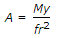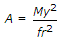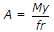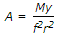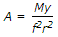Explanation:
No answer description is available. Let's discuss.
Discussion:
4 comments Page 1 of 1.

Sanket said:   2 years ago
Here, I = A * R^2.
(1)

Arifkhan said:   4 years ago
We have: F=My/I.
Also, I=√(I/A)=> I= r^2*A.
Now F =My/r^2*A => A= My/fr^2.
(1)

Chhaya said:   7 years ago
F/Y = M/I,
F = MY/I,
r = sqrt I/A,
I = Ar2.

By substituting the values, you will get the answer.

Kush said:   7 years ago
Pure bending equation:

(F/Ymax) = (M/I) = (E/R).

Put,
Ymax = 2y/2 = y.
I = Ar^2 in the above equation.
Then we get the answer A.